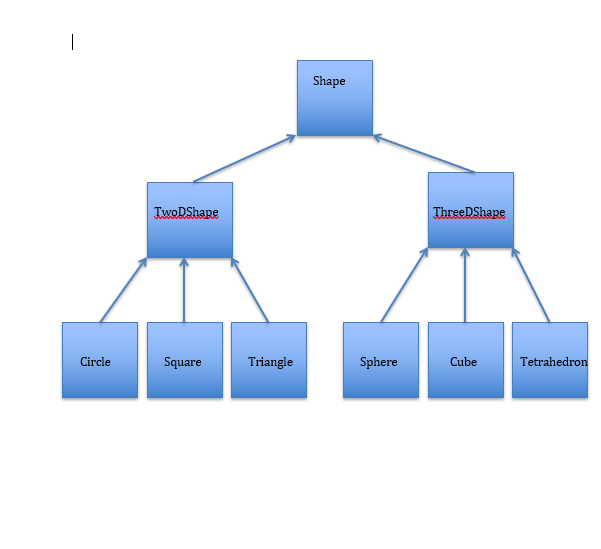# CS 570: Programming Foundations  Programming Assignment #7 Solved

35.00 \$ 17.50 \$

Category:

## Description

Consider the inheritance hierarchy in the figure on the next page. The top of the hierarchy begins with class Shape, which is extended by subclasses TwoDShape and ThreeDShape, corresponding to 2D and 3D shapes, respectively. The third level of this hierarchy contains specific types of 2D and 3D shapes such as circles and spheres, respectively. The arrows in the graph represent isa (inheritance) relationships and the boxes represent classes. For example, a Triangle isa TwoDShape, which in turn isa Shape. Likewise, a Sphere is a ThreeDShape, which in turn is a Shape.

Your task is to implement the entire hierarchy of classes in the figure below. Each two-dimensional shape should contain a getArea() method to calculate the area of the shape. Each three-dimensional shape should contain methods getArea() and getVolume() to calculate the area and volume of the shapes.

Create a program that reads in a set of shape specifications from a text file. It is up to you to design each shape specification. For example, a circle will likely need an (x,y) co-ordinate for its center in addition to the length of the circle’s radius. So in the file you might specify a circle as:

circle 10 20 5

This would be interpreted as a circle object whose center is in co-ordinate (10,20) and has a radius of length 5. The specifications for other shapes are similar in concept. To simplify things your input file may assume that each line in the input text file corresponds to one shape.

The shapes, once read in from the input text file should be stored in an array of Shapes. The program should open an output text file, loop through the Shapes array, and for each shape write to the output file a text description of the object (e.g., circle, square) and its area, and optionally, its volume if it is a three-dimensional shape. Again you may assume that the output file stores one shape per line.